# The Power of Dowsable Energy Fields

by Jeffrey Keen

Jeffrey Keen has a degree in physics from Imperial College, London. He has maintained a passionate interest in physics and scientific research whilst developing a career in industry and business. He has had an orthodox scientific upbringing including the traditional conservative training in physics where the belief is that science is always correct. Accordingly he was dismissive of anything “alternative”. As, for many years, he has been detached from the main stream academic and scientific institutions, coupled with the “wisdom of age” – he is prepared to accept the fact that science does not know everything, and that there may be something worth investigating in the range of “superstitions”, alternative beliefs and therapies, etc., including the very wide subject of dowsing, which he has studied and researched for over twenty years. He is a member of the BSD & EEG.

#### Introduction

To make progress in understanding dowsing, it is necessary to adopt scientific principles which include the use of mathematics, as the universe, in general, has been best understood and defined by numbers. This, in turn, means that scientific progress needs to be made by undertaking laborious, meticulous, re­petitive measurements.

However, attempting a quantitative scientific study of dowsing Earth Energies is not covered in this article, which will become apparent after a brief mention of the vast scope of complex topics being discovered and further investigated by researchers in Earth Energies. As most readers will know, at the largest extreme are two global dowsable grids — the Hartmann and Curry grids that cover the surface of the earth; the former having cosmic connections, whilst the latter probably has terrestrial origins. Then there are earth energy currents that flow river-like across continents, such as one from Israel to Ireland. At the next level down are numerous dowsable energy lines that meander across countries, such as the well-documented Michael & Mary Line from Lands End to Norfolk, or the less well-known Belinus Line which runs from the Isle of Wight to Scotland. A further complexity is that all these lines have positive and negative components, as well as masculine and feminine energies. Superimposed on these dowsable energy lines are constantly changing meteorological effects, and the slower changing effects from geological fault lines and other geological variations in the composition of the earth. Coupled with the above phenomena are the significant impacts of the sun, moon and environment in general. And if this non-exhaustive list is not sufficient, there are the very important effects of underground water flows, which produce dowsable energy lines and spirals.

From this very brief overview, it is apparent that there are many factors making it difficult to understand the basic concepts of dowsing when starting on site to study Earth Energies. In addition, all these concepts interact making the dynamics of dowsing far too complex when attempting to understand the basic physics. The current study of Earth Energies can be likened to a “bottom-up” approach to the understanding of dowsing, and needs complimenting with a “top-down” investigation, which isolates the fundamental physical concepts involved. Hence, one of the purposes of this article is to tie in with some of the observations from Earth Energy studies. The second purpose is to illustrate by use of the following simple analysis, that uses the basic principles of physics (in addition to numerous other examples which due to space limitations are not covered in this article) dowsing, at last, can be put on a firm scientific basis.

The measurements that follow are to a sufficient accuracy to illustrate the principles involved. It is left to other researchers to refine techniques and to undertake the measurements to a higher degree of accuracy with larger samples. Where appropriate, suggestions are made as to areas for future research.

The following assumes a basic knowledge of dowsing and concentrates on the more advanced topics.

Any terminology will be as used in the British Society of Dowsers EEG excellent book of definitions “An Encyclopaedia of Terms”.

#### The Power of Dowsable Energy Fields

One main measurement that can be utilised is Range i.e. the furthest distance a dowsable energy field can be detected from its source. Another measurement relates to the strength of the field, its energy density,  or its power. These terms are synonymous in the dowsing context of this article.

Unlike Range, which is a tangible measurement in mm, Power is best measured using a linear scale marked, say from 0 to 100, which although seeming arbitrary, is, in practice, consistent, as will be demonstrated.

Figures 1 to 9 illustrate the effectiveness of this seemingly non-precise technique. They show that the Power of an object mainly depends on its composition, its mass and, as always, its state of charge.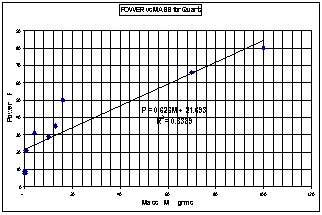Figure 1The Power emitted by 9 small, but different sized, pieces of quartz crystals is plotted against each of their Masses. The straight line on the graph has a high correlation coefficient (R2) giving comfort to the validity of the general conclusion of the Power – Mass relationship, and the formula obtained.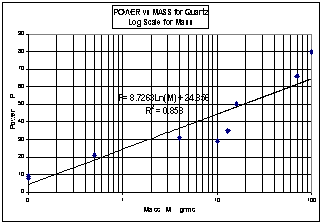Figure 2This graph is exactly the same data as Figure 1, except the M – axis is logarithmic (not linear as in Figure 1). This straight trend line has a slightly higher correlation than Figure 1, and is instinctively satisfactory as the line nearly goes through the origin which illustrates “No mass – no Power”.

Figures 1, 2 and 3 represent three possible trend lines for the same data, which has been restricted to small sized specimens. Interestingly, the highest correlation is for a curvilinear Power–Mass relationship (Figure 3), (which, outside the limited scope of the data, has the unlikely consequence of large Masses tending towards infinite Power!). This suggests an area for further research.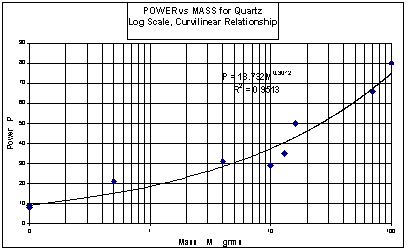Figure 3This is exactly the same data as Figures 1 and 2 and the same logarithmic axis as Figure 2, but the curvilinear fit appears to give a better correlation than the linear trends in Figures 1 and 2.

If instead of using the actual values for the Mass and Power of quartz, the ranked values are used (see graph in Figure 4), the calculation of the Spearman’s coefficient of Rank correlation (Rs), shows a very strong positive correlation of  RS = 0.98.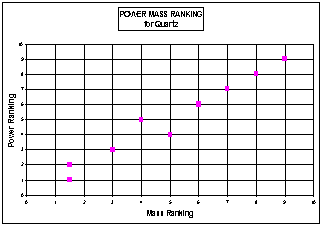Figure 4This is a powerful statistical method of confirming a strong Power-Mass relationship using the calculation of the Spearman’s coefficient of Rank correlation.

These graphs also clearly illustrate that hard igneous crystalline objects, such as quartz, have a greater power than, say, marble, wood, fossils, or clay pots. For example, to achieve a power of 50 requires a wooden log of about 2,700 grams (see Figure 5), or a quartz stone of 16 grams (see Figure 2) .Figure 5This is a similar experiment to Figure 1, but using specimens of wooden logs. The linear graph again has a high correlation between Power and Mass.

Another interesting conclusion from observing the graphs is that, as the Mass tends to zero, the Power tends to a background constant of between 10-20. This is similar to the observations of Range, but this effect cannot so easily be attributed to a zero calibration error, but more probably due to the local background energy, and interactions within the local environment.Figure 6 The data for this graph is more extensive than Figures 1-3, and contains specimens, with much higher Masses as well as numerous different types of crystals and hard igneous rocks. The general Power– Mass relationship in this wider context is illustrated

Figure 6 represents the Power – Mass relationship for different sizes of hard igneous rocks, from small grains to megaliths.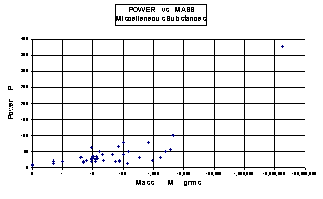Figure 7 The data for this graph contains additional miscellaneous substances to Figure 6, but the Power-Mass relationship, although true in principle, is not as strongly apparent because different substances of the same Mass have different Powers i.e. treating each substance separately as in Figures 1 and 5 is scientifically more rewarding.

Figure 7 includes the data from Figure 6 plus other miscellaneous specimens, which, as expected, does not have such a close relationship as Figure 6 because of the numerous different substances, each having their own dowsing coefficients. These graphs suggest:

##### The Power – Mass Law of Dowsing

which is  a Power-Mass relationship of the form P = j  log M + b  (linear) or P = b.Ml   (curvilinear)

Where P is the Power of the dowsing energy radiated, and M is the Mass of the dowsing emitter. j and l are dowsing coefficients (or constants) for different materials, and b = a local background constant. For quartz, l is approximately equal to 0.3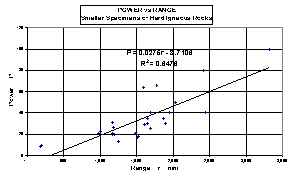Figure 9 The data for Figure 9 is a sub-set of Figure 8, and only relates to smaller specimens of hard igneous rocks.   The correlation of the line is higher than for Figure 8, as the dowsing properties of this sample are more similar than for the miscellaneous samples of Figure 9.

As expected, in general, dowsable objects with a long Range, also have a high Power. This Range-Power relationship has a close relationship that is illustrated in Figure 9 for data with a limited scope, whilst Figure 8 relates to data containing a more extensive Range but the line of which has a different slope caused by one item of data with a high Power and Range. Obviously, more research is required to fine-tune this relationship. In general, these results lead to:

##### The Power – Range  Law of Dowsing

P = q.r + b

Where P is the Power of the dowsing energy radiated, and r is the furthest Range of  detecting the dowsing energy emitted. q is a constant, and b is a constant probably caused by a background dowsing energy.

Although prehistoric man may not have known about logarithms or graphs, he certainly comprehended this Power-Mass law. Somehow, he not only sensed this dowsable Power but was sufficiently motivated to implement it by building such feats as Avebury and Stonehenge. He replaced lower-powered wood henges with as large stone megaliths masses as possible to generate maximum dowsing Power!

Up to now, Power has been a relative measurement because each dowser assigns a perceived value to an arbitrary (but consistent) scale. In other words, a Power of 100 could  represent different dowsing field strengths depending on the person making the observation

To advance the study of the Physics of Power it is necessary to convert the measurement of Power from a relative to an absolute concept, so that all dowsers assign the same numerical value of Power to identical field strengths.

To achieve this, the following definition is suggested.

#### Definition of Power

“The Power of 100 is the field strength produced by a flat face of a cube of pure quartz crystal, having a mass of 100 grams.   (This quartz standard needs to be fully charged, placed in isolation, and not affected by local Earth Energies or local dowsing influences.)”

Individual researchers can then calibrate their own Power scales by using the Power-Mass, or alternatively, the Power-Range Law specified above, and use their own Mass of pure quartz crystal to calculate its Power in comparison to the 100 gram standard.

#### Summary

In summary, the Power of dowsable energy fields is, in general, proportional to the mass and composition of the source. Similarly, the Power of dowsable energy fields is, in general, proportional to the maximum Range of the dowsable energy. Hard igneous rocks have high dowsable Power. Stone-age man clearly understood these three phenomena!  The relatively insignificant advance over the last 5,000 years is that the Power-Mass and Power-Range relationships now have been quantified in both relative and absolute terms, for different substances.

#### Acknowledgements

Thanks are due The Wessex Dowsers, the BSD & the EEG who have helped in opening up the mind of the author to the power of dowsing and its many facets.

Bob Sephton, who has an engineering background, indly reviewed this paper to ensure clarity and accuracy.

Particular thanks are due to Cissie Goldberg, formerly a lecturer in statistics at Sheffield University (Department of Sociology), who checked the validity of the conclusions drawn from the experimental data.

© 2001 Jeffrey S. Keen & BSD EEG